# Ratio

6 numbers are in the ratio 1:5:1:5:5:5. Their sum is 242. What are the numbers?

Result

a =  11
b =  55
c =  11
d =  55
e =  55
f =  55

#### Solution:

$a = \dfrac{ 1 \cdot 242}{ 1+5+1+5+5+5} = 11$
$b = \dfrac{ 5 \cdot 242}{ 1+5+1+5+5+5} = 55$
$c = \dfrac{ 1 \cdot 242}{ 1+5+1+5+5+5} = 11$
$d = \dfrac{ 5 \cdot 242}{ 1+5+1+5+5+5} = 55$
$e = \dfrac{ 5 \cdot 242}{ 1+5+1+5+5+5} = 55$
$f = \dfrac{ 5 \cdot 242}{ 1+5+1+5+5+5} = 55$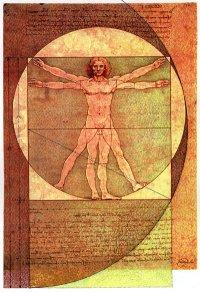Our examples were largely sent or created by pupils and students themselves. Therefore, we would be pleased if you could send us any errors you found, spelling mistakes, or rephasing the example. Thank you!

Leave us a comment of this math problem and its solution (i.e. if it is still somewhat unclear...):Be the first to comment!Tips to related online calculators
Check out our ratio calculator.
Do you have a system of equations and looking for calculator system of linear equations?

#### You need to know the following knowledge to solve this word math problem:

We encourage you to watch this tutorial video on this math problem:

## Next similar math problems:

1. Ratio three numbers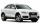Three numbers SUV are in the ratio 1:2:3. Their sum is 24. Find this numbers and write their add and sum.
2. Two numbersFind two numbers whose difference and ratio is 2.
3. RatiosReduce the numbers: 50 in a 1:2 ratio 111 at a ratio of 2:3 70 at 10:50 560 at a ratio of 3:8
4. PeppersIn the box are yellow (a), green (b) and red (c) peppers. Their amount is in a ratio 2:4:1 . Most are yellow peppers and green the least. Calculate the number of peppers each type if the total number of peppers is 70.
5. Moneys in triple ratioMilan, John and Lili have a total 344 euros. Their amounts are in the ratio 1:2:5. Determine how much each of them has?
6. AgesJohn, Teresa, Daniel and Paul have summary 56 years. Their ages are in a ratio of 1:2:5:6. Determine how many years have each of them.
7. Guppies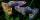Audrey has some guppies in a fish tank. The ration of the oranges guppies to silver guppies is 3:5. She has 12y oranges guppies. Write the number of silver guppies she has in terms of y
8. Ounce749 calories in a 7 - ounce serving . What is the unit rate?
9. Two machinesPerformances of two machines are in a ratio of 7:12. A machine with less power produced 406 pieces of products per shift. a) How many pieces produced per shift second machine? b) How many pieces produced two machines together for five shifts?
10. Money split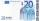Anton and Ferko got 2,500 euros together. The amount was split in 1:4 ratio. How much did each get?
11. Temperature 5Temperature outside starts at 0 Fahrenheit. Over time the temperature changes at a rate of -0.6 Fahrenheit per hour. How long will it take for the temperature to reach -4.5 Fahrenheit?
12. RunnersFor three runners (on the first to third place) is prepared 30 chocolate that they be distributed in the ratio of 3 : 2 : 1 How much chocolate will get everyone?
13. Divide moneyDivide 1200 USD at a ratio of 1:2:3:4:5:6:9:10
14. BuildingAt the building, we divided 240 boards into two piles in a 5: 3 ratio. How many were fewer boards in the lower pile?
15. Calories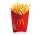A large order of McDonald’s french fries has 500 calories. Of this total, 220 calories are from fat. Find the ratio of the calories from fat to total calories in a large order of McDonald’s french fries.
16. Motor oil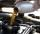30 liters of oil cost 28.80 Euros. How much cost a liter?
17. Pills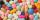If it takes 20 minutes to run a batch of 100 pills how many minutes would it take to run a batch of 50 pills